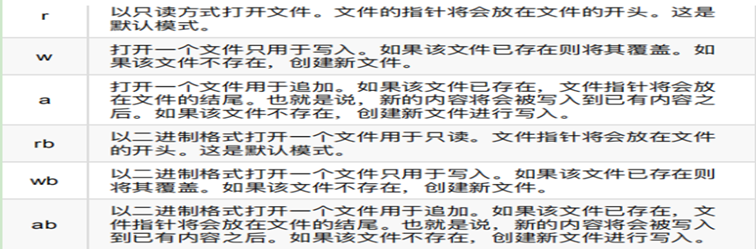2：文件的打开与关闭
3：文件的读写
4：文件及文件夹的相关操作

2：捕获异常
3：异常的传递
4：抛出自定义的异常
5：异常处理中抛出异常

## 一、文件的介绍

### 1 ：什么是文件？image-20210117130855293.png

## 二、文件的打开与关闭

``````打开word软件，新建一个word文件

``````

``````同样，在操作文件的整体过程与使用word编写一份简历的过程是很相似的
``````
• 结论

``````打开文件，或者新建立一个文件

``````

### 2.1 打开文件

``````f = open(‘文件’, 'w')或者f = open('文件', 'r')
``````image-20210117131807308.png

2.1.1 写数据(write)

• 格式

``````对象 = open("文件",w)

``````
• 案例:以写的方式打开文件，写入数据

``````f = open('test.txt', 'w')
f.write('hello world, i am here!')
f.close()
``````
• 继续写入数据

``````f = open('test.txt', 'w')
f.write('I love you')
f.close()
``````
• 总结:

``````如果文件不存在那么创建，如果存在那么就先清空，然后写入数据
``````

• 案例:以读的方式打开文件，读取数据
• 格式

``````对象 = open("文件",r)

print(变量)
``````
• 案例:读取文件（test.txt）

``````f = open('test.txt', 'r')
print(content)
``````
• 总结:

``````如果用open打开文件时，如果使用的"r"，那么可以省略，即只写 open('test.txt')

open(‘test.txt’,”r”, encoding='utf-8')
``````
• 思考：如果只想读取几个字怎么操作?
• 案例:读取指定长度的数据(单位是字节)

``````f = open('test.txt', 'r')
print(content)
``````

• 格式

``````对象 = open("文件",r)

print(变量)
``````
• 案例:读取文件（test.txt）

``````f = open('test.txt', 'r')
print(content)
print(type(content))
``````

• 格式

``````对象 = open("文件",r)

print(变量)
``````
• 案例，一行一行读取

``````f = open('a.txt', 'r', encoding='utf-8')
while True:
if content:
print(content)
else:
break
``````

2.1.5 可写（a）

• 格式

``````对象 = open("",a)

``````
• 案例，在文件中写入数据

``````f = open("test.txt",a)
f.write（"新的数据"）

``````
• 总结:

``````（可写）形式打开文件，如果文件不存在则创建并从头添加写入的内容，存在则原有数据后追加数据
``````

2.1.6 读数据（rb）

• 格式1

``````对象 = open("二进制文件",rb)

print(变量)
``````
• 格式2

``````with open("二进制文件","rb") as 对象:
print(变量)
``````
• 案例:使用尽可能多的方式实现读取图片

``````f = open('33.jpg', 'rb')
print(content)
``````

``````with open("33.jpg","rb") as rf:
print(res)
``````

``````使用习惯：格式2中不需要手动关闭文件，所以经常被使用
``````
• 总结

``````如果没有文件，打开报错，存在该文件才能操作
``````

2.1.7 写数据（wb）

• 格式

``````with open("二进制文件","wb") as 对象:
变量 = 对象.write()
print(变量)
``````
• 案例:备份图片

``````with open("1.jpg","rb") as rf:
with open("textjpg.jpg", "wb") as wf:
res = wf.write(res)
print(res)

``````

### 2.2 关闭文件

• 格式

``````close( )
``````

## 三、文件和文件夹的操作

### 文件的相关操作

3.1 文件重命名

``````os模块中的rename()可以完成对文件的重命名操作
``````
• 格式

``````import os
os.rename("需要修改的文件名","新文件名")
``````
• 案例:

``````import os
os.rename("test.txt","new.txt")
``````

3.2 删除文件

``````os模块中的remove()可以完成对文件的重命名操作
``````
• 格式

``````import os
os.remove("文件名")
``````
• 案例:

``````import os
os.rename("new.txt")
``````

### 文件夹的相关操作

2.1 创建文件夹

``````os模块中的mkdir()可以完成对文件的重命名操作
``````

2.2 获取当前目录

``````os模块中的getcwd()可以获取当前目录
``````

## 四、异常

### 1：什么是异常？

• 演示异常

``````f = open("123.txt","r")     #FileNotFoundError: [Errno 2] No such file or directory: '123.txt'

list =[1,2,3]
print(list)               #IndexError: list index out of range

str="jack"

c= 5/0
print(c)                #ZeroDivisionError: division by zero
``````

### 2 异常处理

2.1 作用

``````捕捉异常可以使用try/except语句。
try/except语句用来检测try语句块中的错误，从而让except语句捕获异常信息并处理。

``````

2.2 语法

``````try:
<语句>        #运行别的代码
except <名字>：
<语句>        #如果在try部份引发了'name'异常
else:
<语句>        #如果没有异常发生
``````
• 代码

``````try:
open("qwe.txt","r")
print("123")
except FileNotFoundError:
print("异常处理")
else:
print("没有异常")
``````

``````try:
open("qwe.txt","r")
print("123")
except FileNotFoundError as result:
print("异常处理",result)
else:
print("没有异常")
``````

2.3：使用except而不带任何异常类型

• 语法

``````try:
正常的操作
except :
发生异常，执行这块代码
else:
如果没有异常执行这块代码
``````
• 代码

``````try:
open("qwe.txt","r")
print("123")
except :
print("异常处理")
else:
print("没有异常")
``````

2.4：使用except而带多种异常类型

• 语法

``````try:
异常的操作
except(Exception1[, Exception2[,...ExceptionN]]]):
发生以上多个异常中的一个，执行这块代码
......................
else:
如果没有异常执行这块代码
``````
• 代码

``````list = [1,2,3,4]
try:
open("qwe.txt", "r")
list
except (NameError,FileNotFoundError) as rese:
print("出现异常")
else:
print("没有异常")
``````

2.5：try-finally 语句

``````try-finally 语句无论是否发生异常都将执行最后的代码
``````
• 语法

``````try:
<语句>
finally:
<语句>    #退出try时总会执行
``````
• 案例

``````try:
fh = open("test.txt", "r")
fh.close()
finally:
print("Error: 没有找到文件或读取文件失败")
``````

finally块中的所有语句执行后，异常被再次触发，并执行except块代码。

2.6 异常的传递

• 代码

``````def func1():
print("---func1--1---")
print(num)
print("---func1--2---")
# def func2():
#     print("--func2--1---")
#     func1()
#     print("--func2--2---")
def func3():
try:
print("---func3--1---")
func1()
print("--func3--2----")
except Exception as result:
print(result)
print("--func3---3---")
func3()
#func2()
``````

2.7:触发异常

• 案例:输入考生的成绩(0~100)

``````def functionName( score ):
if score < 0 or score >100:
raise Exception("Invalid score!", score)
# 触发异常后，后面的代码就不会再执行
functionName(200)
``````

2.8:用户自定义异常

• 定义
通过创建一个新的异常类，程序可以命名它们自己的异常。异常应该是典型的继承自Exception类，通过直接或间接的方式
• 代码：长度不低于3为

``````class ShortInputException(Exception):
def __init__(self, length, atleast):
self.length = length
self.atleast = atleast
def main():
try:
s = input('请输入 --> ')
if len(s) < 3:
# raise引发一个你定义的异常
raise ShortInputException(len(s), 3)
except ShortInputException as result:#x这个变量被绑定到了错误的实例
print('ShortInputException: 输入的长度是 %d,长度至少应是 %d'% (result.length, result.atleast))
else:
print('没有异常发生')
main()
``````

## 五、模块

``````Python 模块(Module)，是一个Python文件，以.py 结尾，包含了Python 对象定义和Python语句
``````
• 下例是个简单的模块 aa.py：

``````def test1():
print("我是模块1")
def test2():
print("我是模块2")
``````

5.1:模块的引入

5.1.1：import

``````import module1[, module2[,... moduleN]]
``````

test.py 文件代码

``````import aa
aa.test1()
aa.test2()
#我是模块1
#我是模块2
``````

• 代码:开平方根

``````import math
print(math.sqrt(4))
#2
``````

``````注意:一个模块只会被导入一次，不管你执行了多少次。这样可以防止导入模块被一遍又一遍地执行。
``````

5.1.2:from…import 语句

Python 的 from 语句让你从模块中导入一个指定的部分到当前命名空间中，语法如下

``````from modname import name1[, name2[, ... nameN]]
``````

test.py 文件代码

``````from aa import test1
test1()
#我是模块1
``````

``````from aa import test1,test2
test1()
test2()
#我是模块1
#我是模块2
``````
• 思考：如果需要导入模块中的部分有很多，怎么操作?

5.1.3:from…import * 语句

``````from modname import *
``````

test.py 文件代码

``````from aa import *
test1()
test2()
#我是模块1
#我是模块2
``````
• 扩展

``````使用__all__魔幻方法

``````

aa.py

``````__all__=('test1','test2')
def test1():
print("我是模块1")
def test2():
print("我是模块2")
def test3():
print("我是模块2")
``````

test.py

``````from aa import *
test1()
test2()
``````

## 六、Python中的包

``````test.py
day
|-- __init__.py
|-- runoob1.py
|-- runoob2.py
``````
• 源代码如下：
day/runoob1.py

``````def runoob1():
print "I'm in runoob1"
``````

day/runoob2.py

``````def runoob2():
print "I'm in runoob2"
``````

``````# 导入 Phone 包
from day.runoob1 import runoob1
from day.runoob2 import runoob2
runoob1()
runoob2()
``````
• 结果

``````I'm in runoob1
I'm in runoob2
``````

### Hibernate之对象关系映射_空城1995的博客-程序员秘密

MAC 架构RRC 控制 MAC 配置。MAC 实体UE 的 MAC 实体处理以下传输信道：广播信道（BCH）;下行链路共享信道（DL-SCH）;寻呼信道（PCH）;上行链路共享信道（UL-SCH）;随机接入信道（RACH）。当 UE 配置有 SCG 时，向 UE 配置两个 MAC 实体：一个用于 MCG，一个用于 SCG。除非另有说明，否则 UE 中的不同 MAC 实体的功......

### void* 转 int_qt void* 转int_Yfw&武的博客-程序员秘密

int a = 10; //测试数据int b;void* p = &amp;a; //void类型的指针指向 a这个地址b = (int)p; //从p这个地址读取int字节大小的数据（取得int类型的数据）

### Ros点云格式转换总结_waymo数据集 点云转ros_扛着相机的翻译官的博客-程序员秘密

Ros点云格式转换总结三种格式:sensor_msgs::PointCloudsensor_msgs::PointCloud2pcl::PointCloud&lt;T&gt;其中，PointCloud2和pcl::PointCloud可以相互转换，PointCloud和PointCloud2可以相互转换，PointCloud和pcl::PointCloud的转换需要使用PointCloud2中转。PointCloud⟺PointCloud2⟺pcl::PointCloud&lt;T&gt;Po

### Alluxio架构与数据流_气运2020的博客-程序员秘密

Alluxio架构与数据流Alluxio：架构及数据流 - 简书 (jianshu.com)1 架构1.1 概述​ Alluxio作为大数据和机器学习生态系统中的一个新的数据访问层，配置在任何持久性存储系统(如Amazon S3、Microsoft Azure对象存储、Apache HDFS或OpenStack Swift)和计算框架(如Apache Spark、Presto或Hadoop MapReduce)之间。**请注意，Alluxio不是一个持久化存储系统。**使用Alluxio作